# Tangent

Tangent

The word "tangent" comes from the Latin word tangere, which means "to touch".

In geometry, the tangent line (or tangent) means a line or plane that intersects a curved line or surface at exactly one point.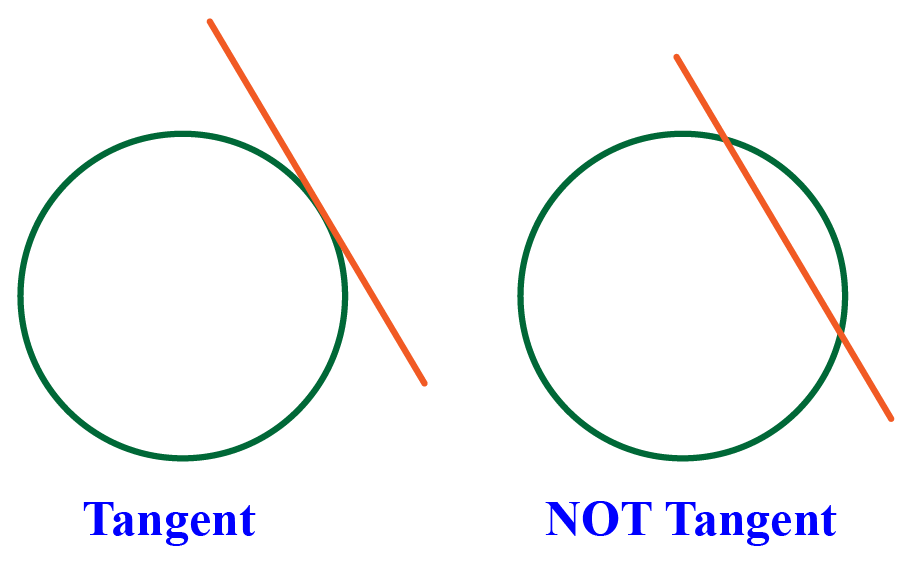The word "tangent" can also mean the trigonometric function.

Here, we shall learn about the tangent definition in geometry, tangent to a circle, and tangent line equation as we scroll down.

Let's get going!

## Lesson Plan

 1 What Is the Meaning of Tangent? 2 Challenging Questions on Tangent 3 Important Notes on Tangent 4 Solved Examples on Tangent 5 Interactive Questions on Tangent

## What Is the Meaning of Tangent?

### Tangent in Geometry (Definition)

Tangent line or tangent in geometry means a line or plane that intersects a curve or a curved surface at exactly one point.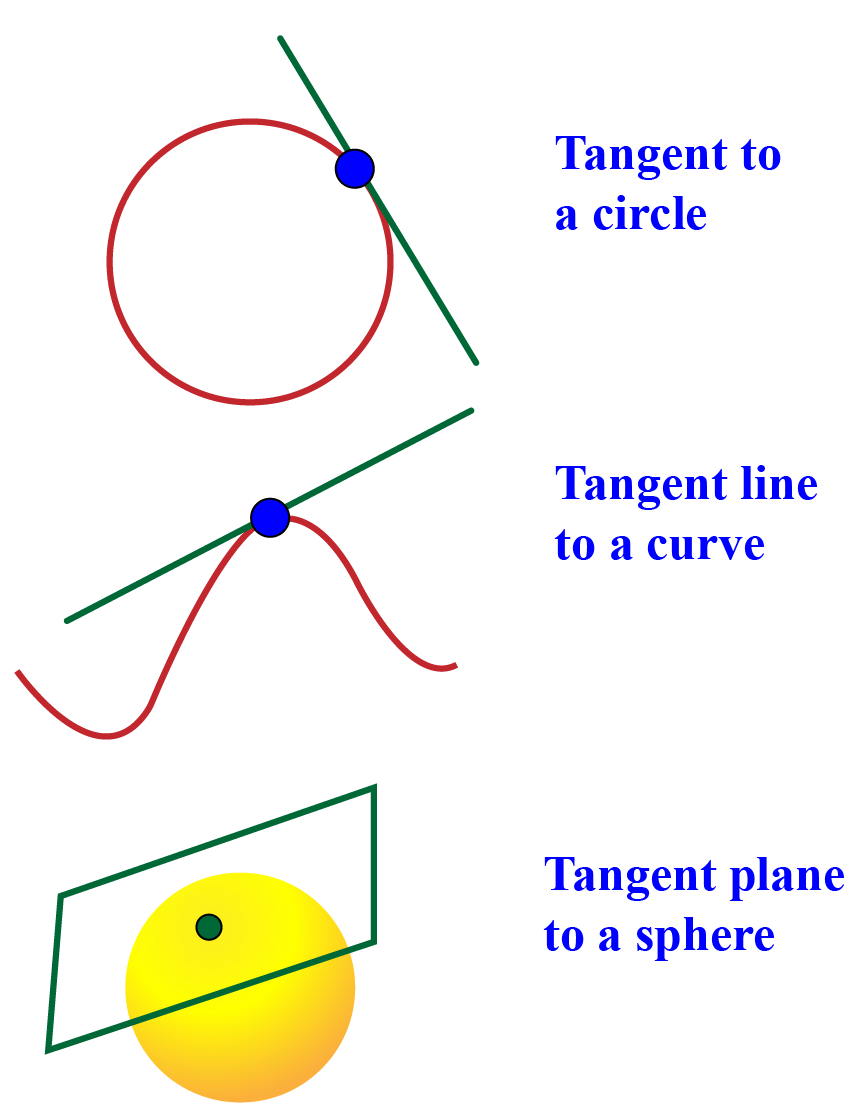## How to Find the Equation of a Tangent Line?

We are familiar that the "point-slope form of a line" through point $$(x_0,y_0)$$ is

$y-y_0 = m(x-x_0)$

To find the equation of the tangent line to a curve (function), we need its slope and a point through which it passes.

The slope of the tangent line is equal to the derivative of the function evaluated at the given point of contact.

Why is that so? The idea behind this is that the instantaneous rate of change of $$y$$ with respect to $$x$$ (which is given by the derivative of $$y$$ with respect to $$x$$ at the given point of contact) represents the slope of the tangent line.

Example

For a curve $$y=f(x)$$ containing the point $$(x_1,y_1)$$, the equation of the tangent line to the curve at $$(x_1,y_1)$$ is given by $$y-y_1=f'(x_1)(x-x_1)$$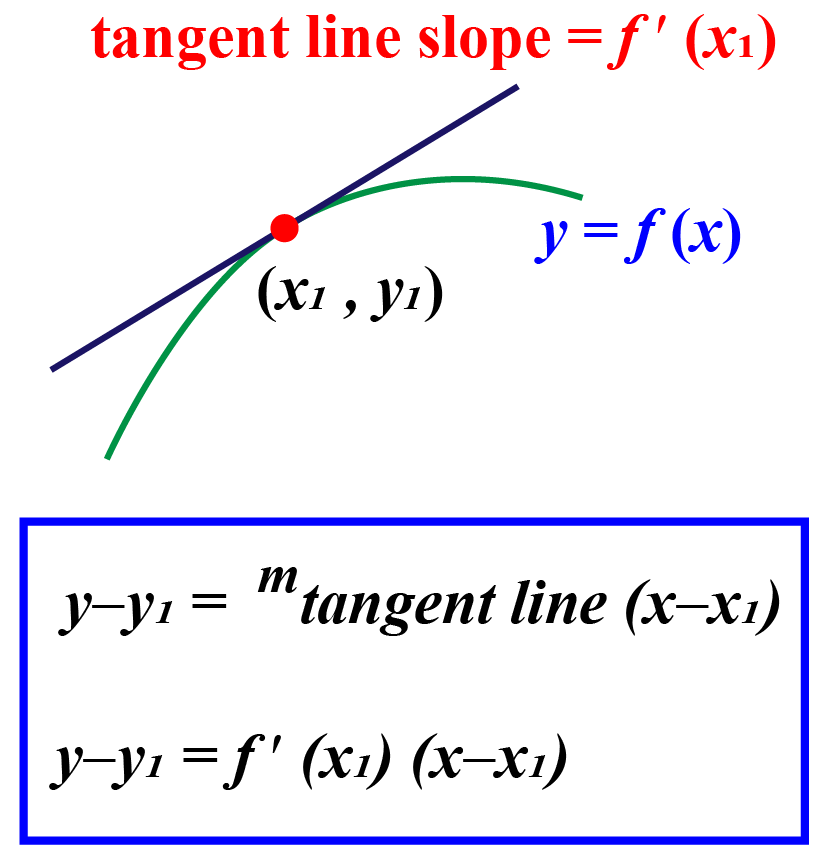To sum up, the steps to find the equation of a tangent line to a curve at a specific point are:
1) Find the first derivative of $$f(x).$$
2) Plug the $$x_1$$-value of the given point into $$f '(x_1)$$ to find the slope at $$x_1.$$
3) Plug $$x_1$$ value into $$f(x)$$ to find the $$y$$ coordinate of the tangent point.
4) Use the slope from step $$2$$ and point from step $$3$$ using the point-slope formula to write the equation for the tangent line.

### Example

Point $$(1,5)$$ lies on a curve given by $$y=f(x)=x^3-x+5$$. Find the equation of the tangent line to the curve that passes through the given point.

To write the equation of a line we need two things:

1. Slope

2. A point on the line

It is given that the curve contains a point $$(1,5)$$

The slope is the same as the slope of the curve at $$x=1$$ which is equal to the function’s derivative at that point:

\begin{align*} f(x)&=x^3-x+5\\ f'(x)&=3x^2 - 1\\f'(x)&=3(1)^2 - 1=2\end{align*}

Substituting the slope $$m$$ in the point-slope form of the line we would have:

\begin{align*} y-y_0 &= m_{tangent}(x-x_0)\\ y-5 &=2(x-1)\\ \end{align*}

Converting the above equation into $$y$$-intercept form as:

\begin{align*} y-5 &=2(x-1)\\ y-5&=2x-2\\ y&=2x+3\end{align*}

$$\therefore$$ The equation for the tangent line is $$y=2x-13$$

## How to Find the Slope of the Tangent Line?

The idea behind finding the slope of the tangent at a particular point is to find the instantaneous rate of change of $$y$$ with respect to $$x$$ of two very closely located points.

This is given by the derivative of $$y$$ with respect to $$x$$ at $$x_1$$; the point of tangency.

The slope of the tangent line is very close to the slope of the line through $$(x_1, f(x_1))$$ and a nearby point on the graph, for example $$(x_1 + h, f(x_1 + h))$$.

These lines are called secant lines.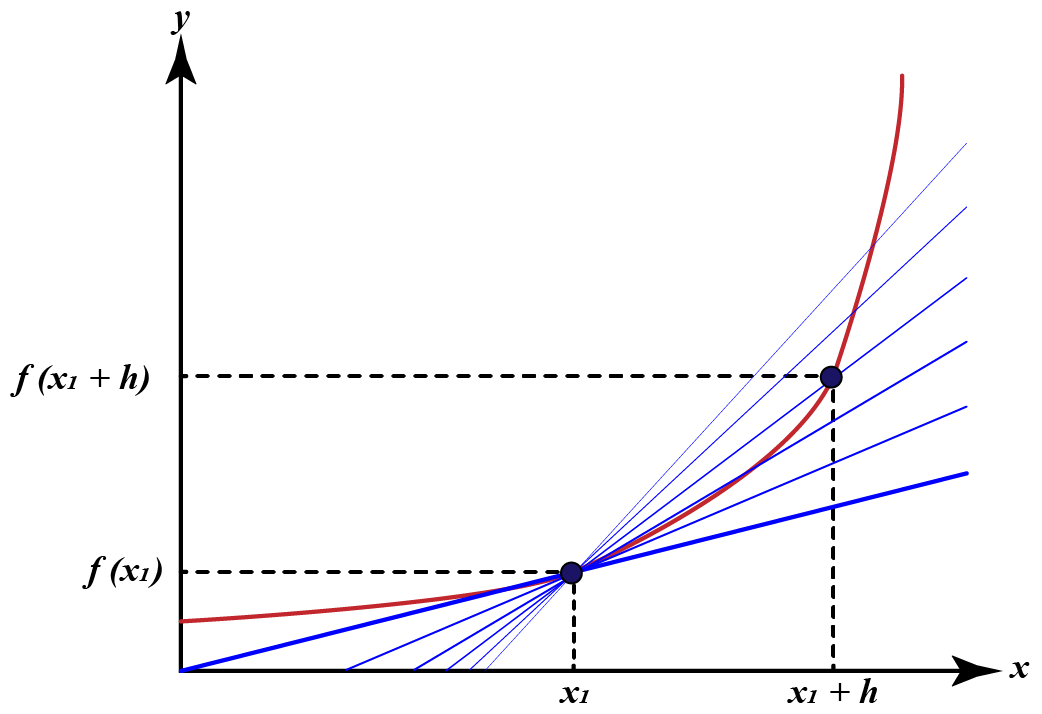A value of $$h$$ close to zero gives the close approximation to the slope of the tangent line, and smaller values (in absolute value) of $$h$$ will, in general, give better approximations.

The secant's line slope is the difference between the $$y$$ values of these points divided by the difference between the $$x$$ values, that is,

$m=\dfrac {\Delta f(x_1)}{\Delta x_1}=\dfrac {f(x_1+h)-f(x_1)}{(x_1+h)-(x_1)}=\dfrac {f(x_1+h)-f(x_1)}{h}$

$f'(a)=\lim _{h\to 0}{\frac {f(x_1+h)-f(x_1)}{h}}$

## Tangent and Normal Line to a Curve

The normal line to a curve at a specified point is the line through that point and perpendicular to the tangent.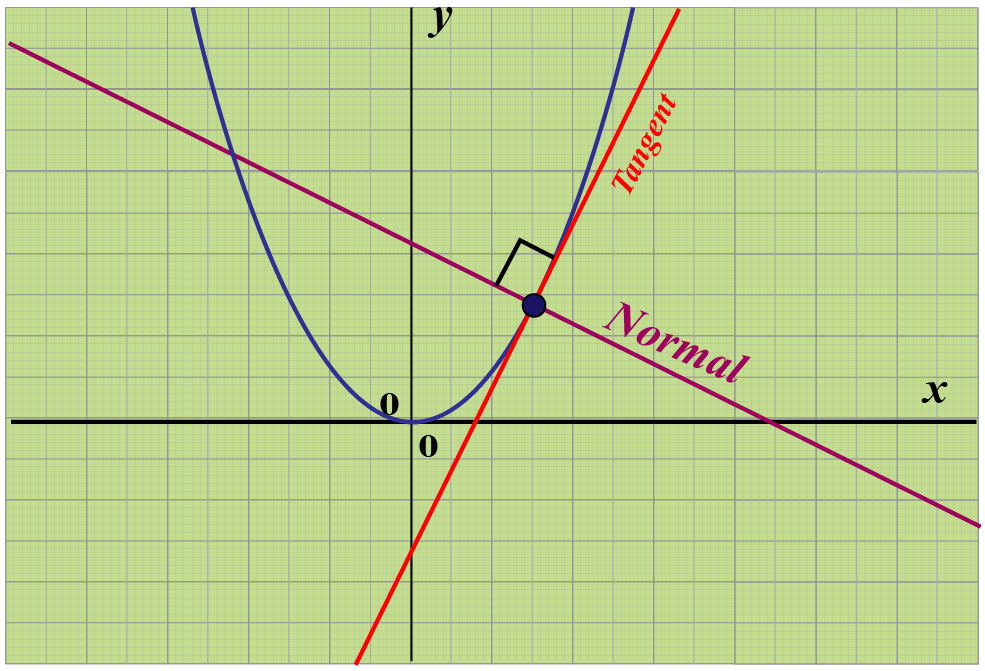Recall that the parallel lines have the same slopes and the slopes of perpendicular lines are the negative reciprocals of each other. If $$m_1$$ and $$m_2$$ are the slopes of two perpendicular lines

Then, $m_2 = - \dfrac{1}{m_1}$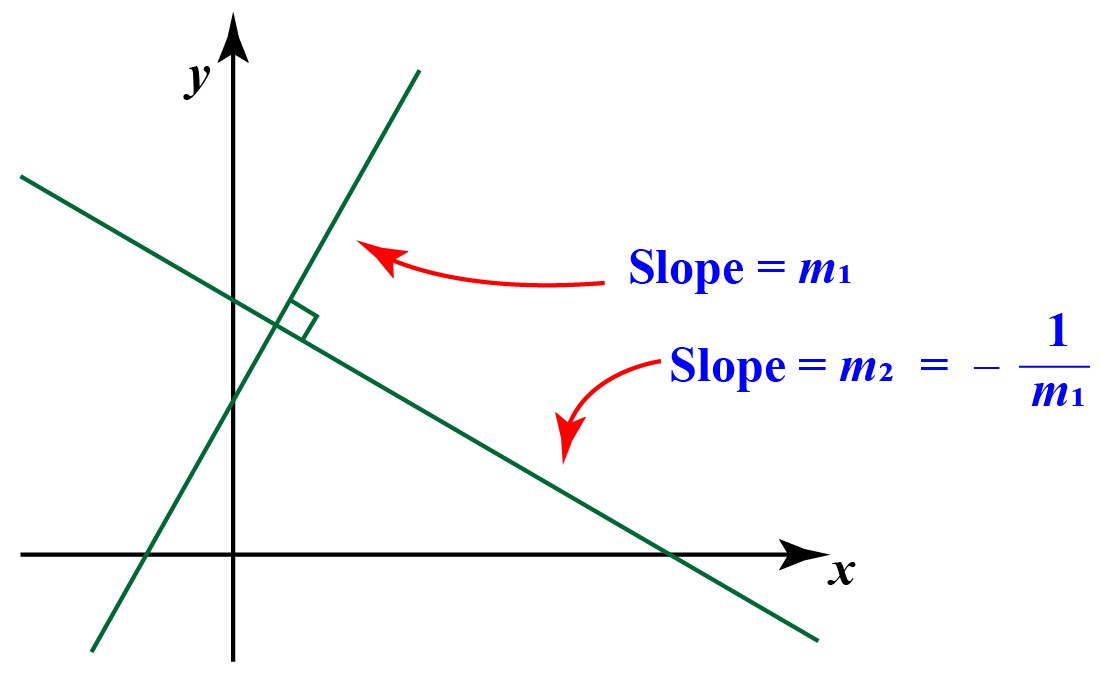We want the slope of the line that is perpendicular to the curve at a point, that is perpendicular to the tangent line to the curve at that point $$x_1$$:

\begin{aligned}
m_{\text {normal line }} &=\frac{-1}{m_{\text {tangent line }}} \\
&=\frac{-1}{f^{\prime}\left(x_{1}\right)}
\end{aligned}

Hence, we can write the equation for the normal line at $$(x_1, y_1)$$ as:

\begin{array}{l}
y-y_{1}=m_{\text {normal line }}\left(x-x_{1}\right) \\
y-y_{1}=\dfrac{-1}{f^{\prime}\left(x_{1}\right)}\left(x-x_{1}\right)
\end{array}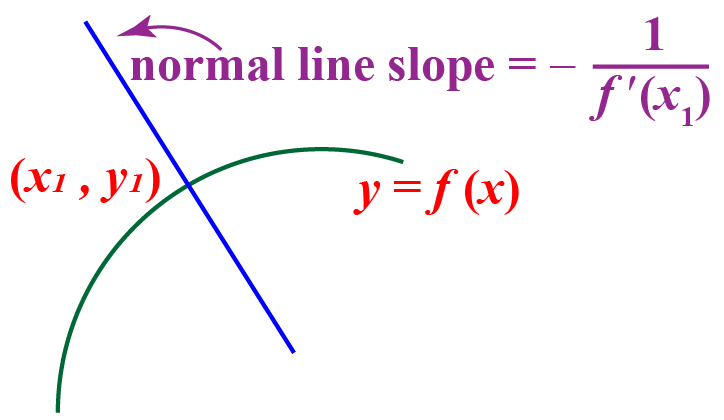Challenging Questions
• Find the equation of the line normal to the curve at the point (1, 8) for the curve given by $$y = f(x) = x^2 - x + 9$$.
• What will be the equation of the line which contains the point (1,2) and is parallel to the line through the points (0,1)?

## Tangent Line Calculator

The steps to use the below tangent line calculator are:

Step 1: Enter the equation of the curve and $$x$$ value in the given input field.

Step 2: Press “Calculate” to get the output.

Step 3: The slope of the tangent line and the equation of the tangent line will be displayed.

## Solved Examples

 Example 1

A function $$g$$ is such that, $$g'(5)=2$$ and $$g(5)=-3$$. What will be the equation for the tangent line to $$g$$ at $$x=5$$.

Solution

Given $$g'(5)=2$$

This means that the slope of the tangent at $$x=5$$ is $$m=2$$

The tangent line passes through the point $$(2,-3)$$

Using the point-slope form of the line we would have:

\begin{align*} y-y_0 &= m(x-x_0)\\ y-(-3) &=2(x-5)\\ y+3 &=2(x-5)\end{align*}

We can also convert the above equation into $$y$$-intercept form as:

\begin{align*} y+3 &= 2x-10\\ y&=2x-10-3\\ y&=2x-13\end{align*}

 $$\therefore$$ The equation for the tangent line is $$y=2x-13$$
 Example 2

Point $$(1,5)$$ lies on a curve given by $$y=f(x)=x^3-x+5$$. Find the equation of the tangent line to the curve that passes through the given point.

Solution

To write the equation of a line we need two things:

1. Slope

2. A point on the line

It is given that the curve contains a point $$(1,5)$$

The slope is the same as the slope of the curve at $$x=1$$ which is equal to the function’s derivative at that point:

\begin{align*} f(x)&=x^3-x+5\\ f'(x)&=3x^2 - 1\\f'(x)&=3(1)^2 - 1=2\end{align*}

Substituting the slope $$m$$ in the point-slope form of the line we would have:

\begin{align*} y-y_0 &= m_{tangent}(x-x_0)\\ y-5 &=2(x-1)\\ \end{align*}

Converting the above equation into $$y$$-intercept form as:

\begin{align*} y-5 &=2(x-1)\\ y-5&=2x-2\\ y&=2x+3\end{align*}

 $$\therefore$$ The equation for the tangent line is $$y=2x+3$$
 Example 3

Jamie is asked to find the equation for both the tangent line to the curve $$y=x^3$$ at $$x_1=1$$. Can you help him?

Solution

First, we find the derivative:

$$y^{\prime}=f^{\prime}(x)=\left(x^{3}\right)^{\prime}=3 x^{2}$$

The value of the derivative at the point of interest is:

$$f^{\prime}\left(x_{0}\right)=3 \cdot 1^{2}=3$$

Calculating $$y_0$$:

$$y_{0}=\left(x_{0}\right)^{3}=1^{3}=1$$
Substitute this in the equation of tangent:
$$\begin{array}{l} y-1=3(x-1) \\ y-1=3 x-3 \\ y=3 x-2 \end{array}$$

 $$\therefore$$ The equation for the tangent line is $$y=3x-2$$

## Interactive Questions

Here are a few activities for you to practice. Select/Type your answer and click the "Check Answer" button to see the result.

## Let's Summarize

The mini-lesson targeted the fascinating concept of a tangent. We also learned tangent definition, tangent geometry, tangent to a circle, tangent line equation, and checked out tangent line calculator. The math journey around set builder notation starts with what a student already knows, and goes on to creatively crafting a fresh concept in the young minds. Done in a way that not only it is relatable and easy to grasp, but also will stay with them forever. Here lies the magic with Cuemath.

At Cuemath, our team of math experts is dedicated to making learning fun for our favorite readers, the students!

Through an interactive and engaging learning-teaching-learning approach, the teachers explore all angles of a topic.

Be it worksheets, online classes, doubt sessions, or any other form of relation, it’s the logical thinking and smart learning approach that we, at Cuemath, believe in.

How do you find tangent in geometry?

In geometry, to find the tangent line (or tangent) we look for a line or plane that intersects a curved line or surface at exactly one point.

What is the tangent equation?

For a curve $$y=f(x)$$ containing the point $$(x_1,y_1)$$, the equation of the tangent line to the curve at $$(x_1,y_1)$$ is given by $$y-y_1=f'(x_1)(x-x_1)$$

What do you mean by tangent circles?

In geometry, tangent circles are circles in a common plane that meet in only a single point.

What is the equation of tangent and normal?

The slopes of perpendicular lines are the negative reciprocals of each other.

\begin{aligned}
m_{\text {normal line }} &=\frac{-1}{m_{\text {tangent line }}} \\
&=\frac{-1}{f^{\prime}\left(x_{0}\right)}
\end{aligned}

Hence we can write the equation for the normal line at $$(x_1, y_1)$$ as

\begin{array}{l}
y-y_{1}=m_{\text {normal line }}\left(x-x_{1}\right) \\
y-y_{1}=\frac{-1}{f^{\prime}\left(x_{1}\right)}\left(x-x_{1}\right)
\end{array}

What is the slope of tangent?

The slope of the tangent line at a point on the function is equal to the derivative of the function at the point of tangency.

For a curve $$y=f(x)$$ containing the point $$(x_1,y_1)$$, the equation of the tangent line to the curve at $$(x_1,y_1)$$ is given by $$y-y_1=f'(x_1)(x-x_1)$$

Circles and Tangents
grade 10 | Questions Set 1
Circles and Tangents
Circles and Tangents
grade 10 | Questions Set 2
Circles and Tangents
Circles and Tangents
grade 10 | Questions Set 1
Circles and Tangents